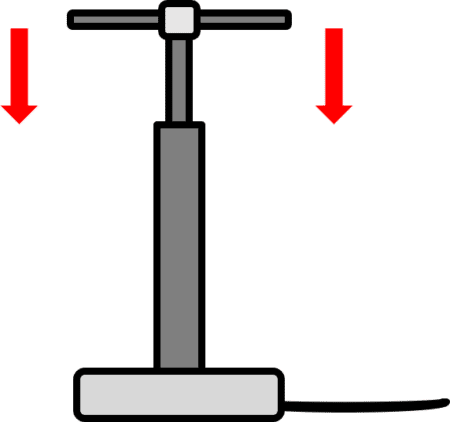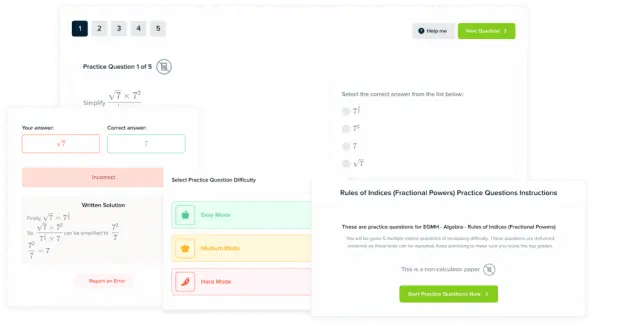# Gases and Pressure

GCSEAQACombined Science FoundationCombined Science HigherPhysics FoundationPhysics Higher

## Gases and Pressure

The pressure, temperature, volume and kinetic energy of a gas and it’s particles are all related. The particle model explains these relationships and how changing one variable might affect another.

## Temperature and Pressure

Temperature and Pressure in a gas are directly related. Particles in a gas are always moving around, in random directions with random speeds. When the temperature of the gas is increased, the following things happen:

• Because temperature is increased, there is a transfer from heat energy to kinetic energy in the particles.
• Kinetic energy and speed are related by $\textcolor{aa57ff}{K = \dfrac{1}{2} mv^2}$, so when the kinetic energy increases so does the speed of the particles.
• Therefore, the average speed of the particles increases with the temperature.
• When the gas particles are moving with increased speed, there are more collisions between them. They also collide with the walls of the sealed container they’re in.
• The pressure exerted by the gas is equal to the total force of all the individual particles on the area of the container, because $\textcolor{aa57ff}{p = \dfrac{F}{A}}$.
• Therefore, an increase in temperature leads to an increase in the kinetic energy and speed of the particles, leading to an increase in pressure of the gas.
• This is only true however if the volume of the gas stays constant.
GCSECombined Science FoundationCombined Science HigherPhysics FoundationPhysics HigherAQA

## Pressure and Volume

If the temperature of the gas is constant, pressure and volume are inversely proportional. This means as one increases, the other decreases. For example, if you were to increase the volume that a gas occupies, it’s pressure would decrease. This is because the particles are spaced further apart in a larger volume, so collisions with the walls of the container are less common.

To show that pressure and volume are inversely proportional, we can write:

$\textcolor{f21cc2}{pV = \text{constant}}$

• $\textcolor{f21cc2}{p}$ is the pressure in pascals $\left(\text{Pa}\right)$.
• $\textcolor{f21cc2}{V}$ is the volume in metres cubed $\left(\text{m}^3\right)$.

For example, if the volume of a gas (kept at a constant temperature) is doubled, it’s pressure must have halved in order for $\textcolor{f21cc2}{pV}$ to stay constant.

GCSEPhysics FoundationPhysics HigherAQA

## Increasing the Pressure of a GasIf energy is transferred by a force, this is called work. Doing work on a gas increases the internal energy of the gas, which increases it’s temperature.

A bike pump is an example of doing work on a gas. The pressure of the gas means that the gas exerts a force on the lever of the bike pump. Work is done against this force by your arms to push the lever down and pump the tires.  This increases the internal energy and hence the tire should get warmer the more you pump it.

GCSEPhysics HigherAQA

## Gases and Pressure Example Questions

• When the temperature of the gas increases, there is an increase in kinetic energy of the particles in the gas.
• The particles now move at a higher average speed. This means there is more collisions between the particles and between the walls of the container.
• The force on the walls of the container increases, and pressure of the gas increases as a result.Gold Standard Education

Any 1 from:

• $\bold{pV = \text{constant}}$
• Pressure and volume are inversely proportional.Gold Standard Education

Energy is transferred by a force when work is done on a gas. The internal energy increases as a result, which leads to a temperature increase.Gold Standard Education

## Gases and Pressure Worksheet and Example Questions

### Particle Model and Pressure Questions

GCSEOfficial MMEProduct